2022-09-2100

## 2 内容概要

### 神经网络中的神经元和突触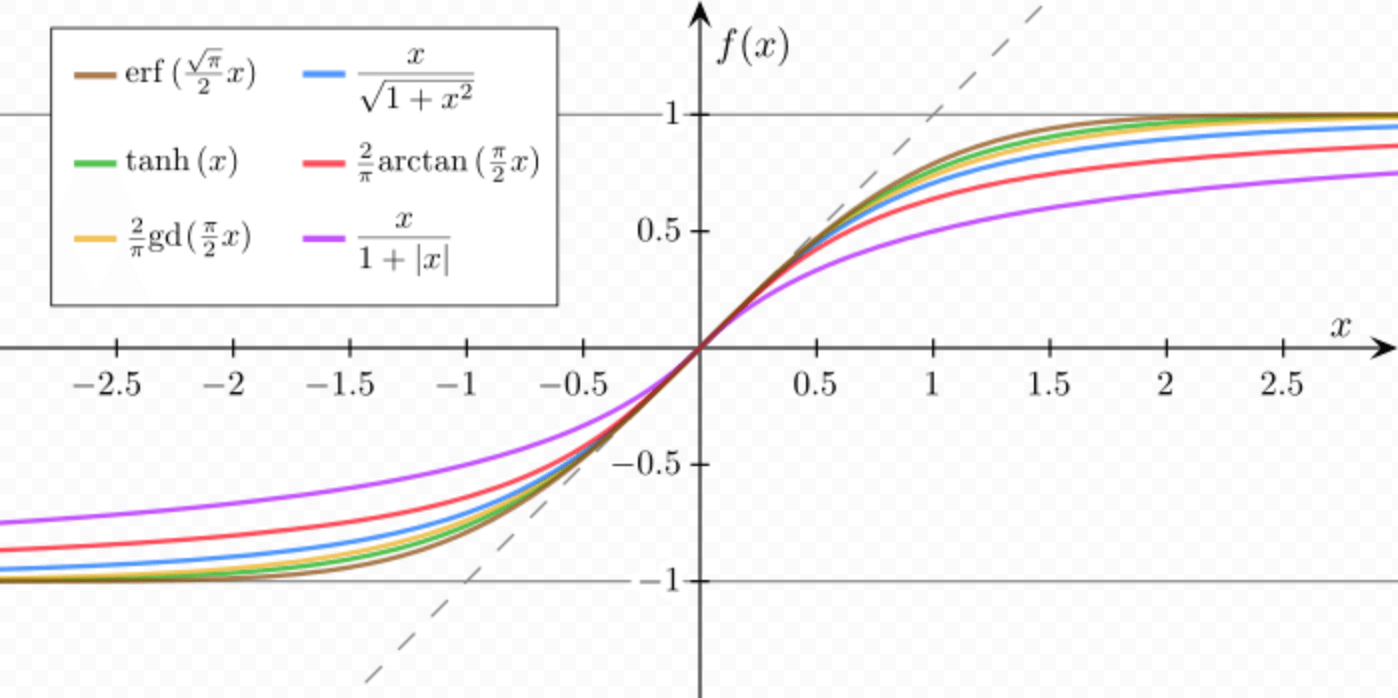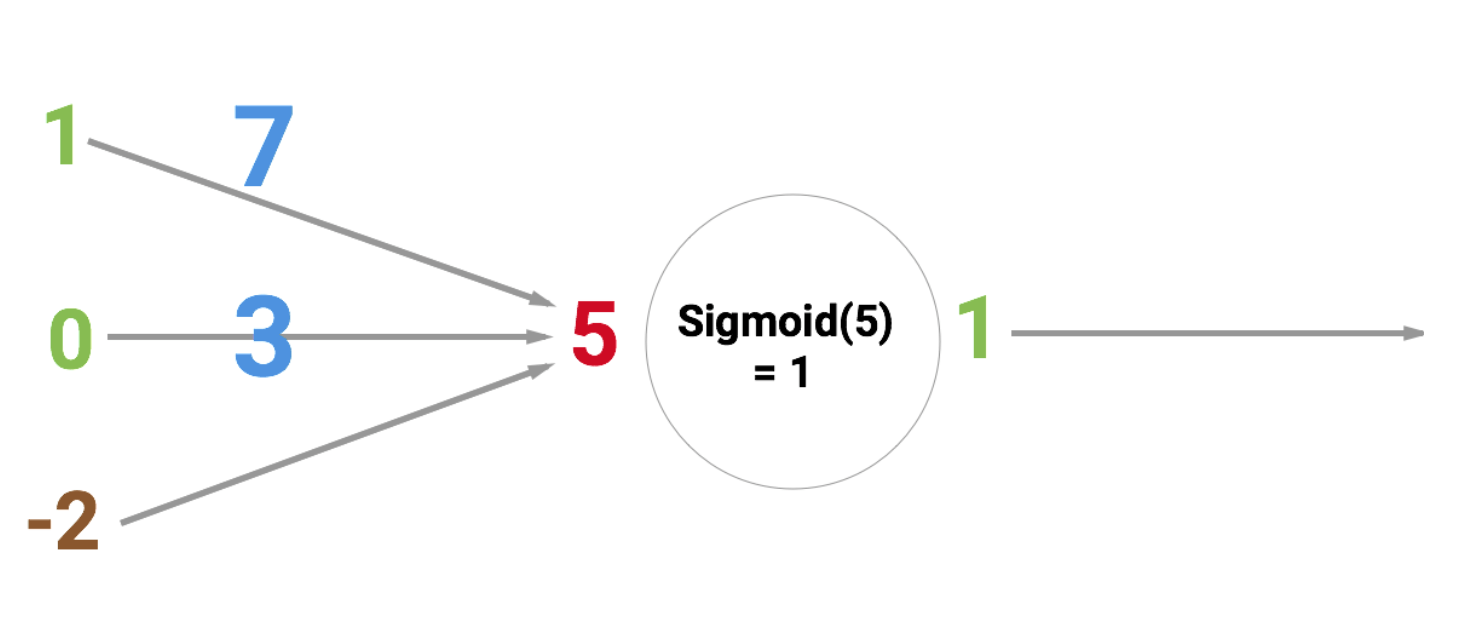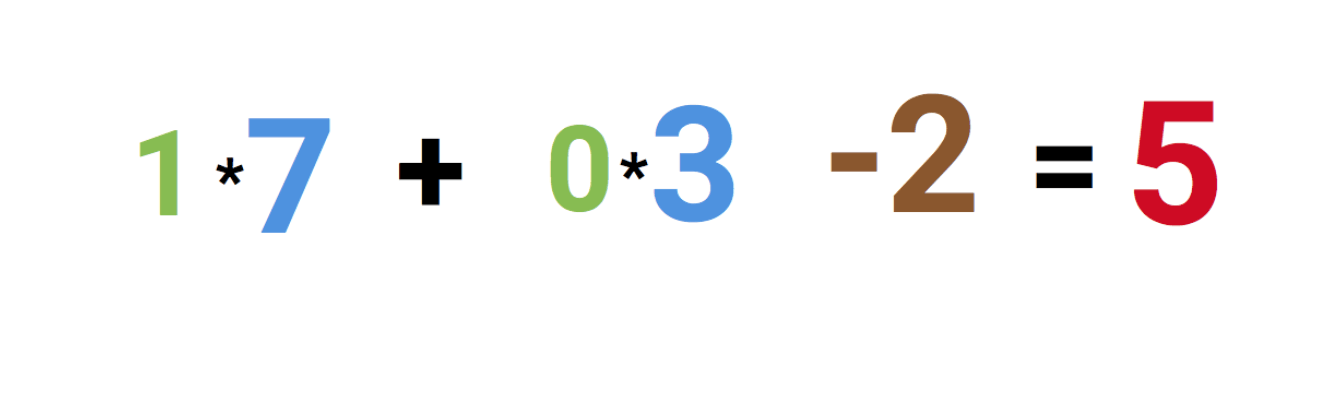### 如何构建神经网络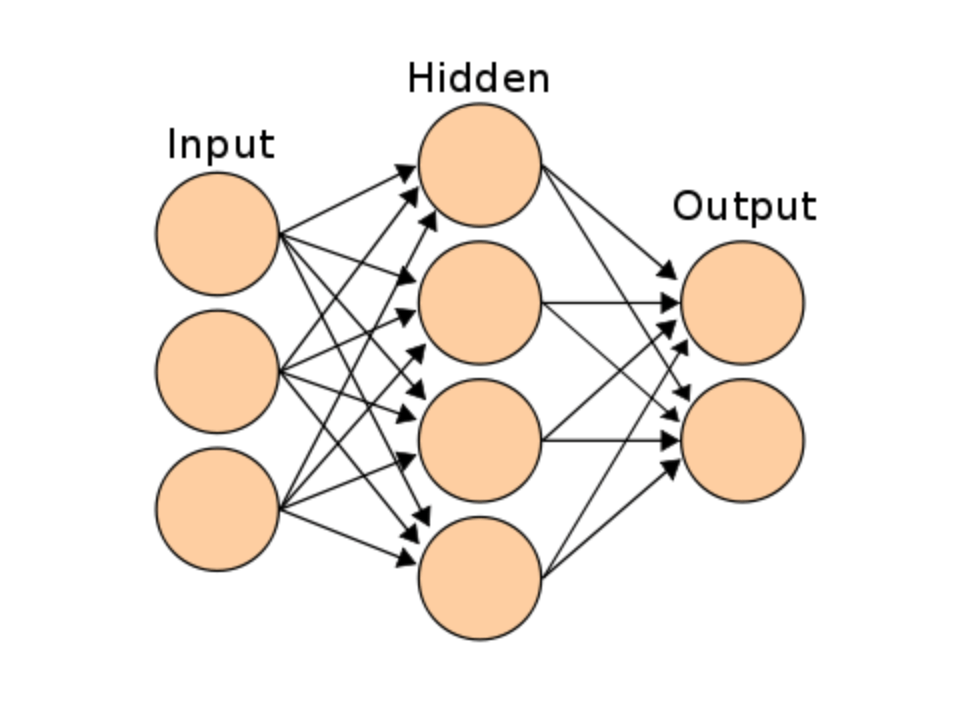### 代码实现

``````const { Layer, Network } = window.synaptic;
var inputLayer = new Layer(2);
var hiddenLayer = new Layer(3);
var outputLayer = new Layer(1);

inputLayer.project(hiddenLayer);
hiddenLayer.project(outputLayer);
var myNetwork = new Network({
input: inputLayer,
hidden: [hiddenLayer],
output: outputLayer
});

// train the network - learn XOR
var learningRate = .3;
for (var i = 0; i < 20000; i++) {
// 0,0 => 0
myNetwork.activate([0,0]);
myNetwork.propagate(learningRate, );
// 0,1 => 1
myNetwork.activate([0,1]);
myNetwork.propagate(learningRate, );
// 1,0 => 1
myNetwork.activate([1,0]);
myNetwork.propagate(learningRate, );
// 1,1 => 0
myNetwork.activate([1,1]);
myNetwork.propagate(learningRate, );
}``````

``````console.log(myNetwork.activate([0,0]));
-> [0.015020775950893527]
console.log(myNetwork.activate([0,1]));
->[0.9815816381088985]
console.log(myNetwork.activate([1,0]));
-> [0.9871822457132193]
console.log(myNetwork.activate([1,1]));
-> [0.012950087641929467]``````

## 3 精读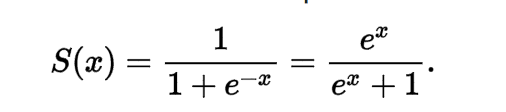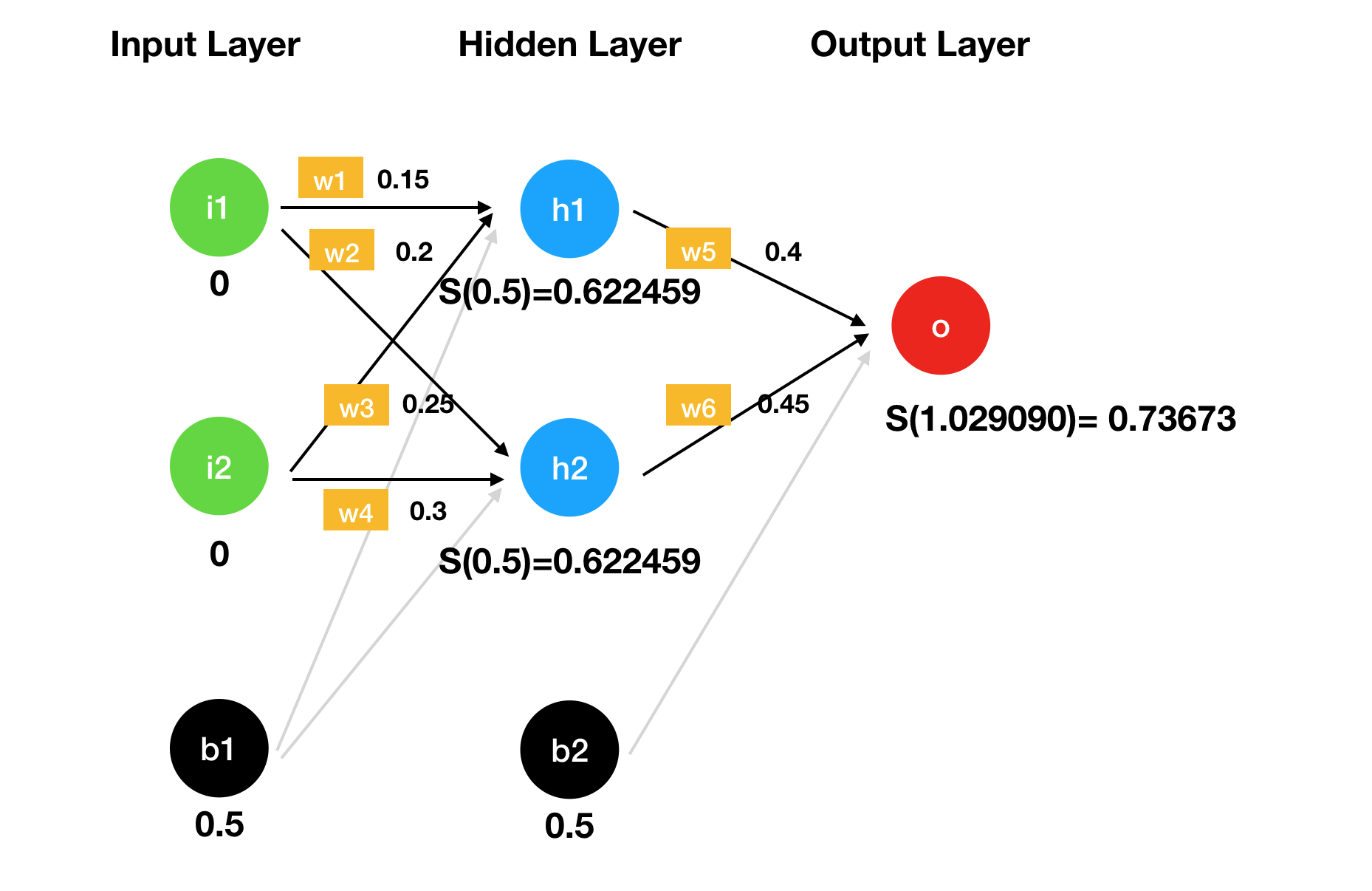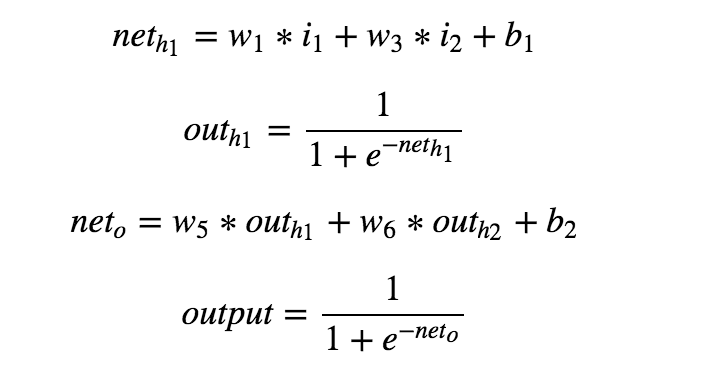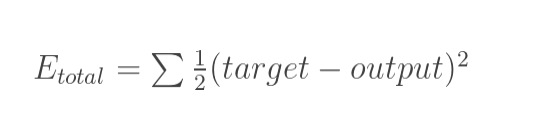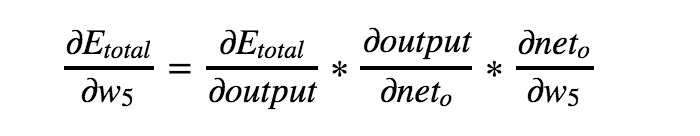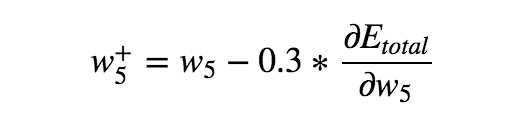## 4. 总结

### 相关资料

1. A Step by Step Backpropagation Example — by Matt Mazur

2. Hackers Guide to Neural Nets — by Andrej Karpathy

3. NeuralNetworksAndDeepLarning — by Michael Nielsen

4. Synaptic.js